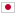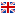Contest Duration: - (local time) (180 minutes) Back to Home
J - XORAND/Time Limit: 4 sec / Memory Limit: 256 MB

### Problem

Yu loves bitwise AND operation and big number. Yu's friend Yihuo loves bitwise XOR operation and small number. They are good friends that whenever someone sends them an array of numbers as a gift, they share the numbers with each other.

For an array of non-negative integers p_1, p_2, ..., p_k, let D be the result of bitwise AND between all numbers in the array (p_1\rm{\ AND\ }p_2\rm{\ AND\ }...\rm{\ AND\ }p_k), and let X be the result of bitwise XOR between all numbers in the array (p_1\rm{\ XOR\ }p_2\rm{\ XOR\ }...\rm{\ XOR\ }p_k). Yu's satisfaction value for p_1, p_2, ..., p_k is D, Yihuo's satisfaction value for the same array of numbers is -X (Note that Yihuo loves small number. The less X is, the more Yihuo satisfies.)

Whenever they receive an array of non-negative integer p_1, p_2, ..., p_k as a gift, they cut the array in the middle and Yu gets the former part, Yihuo gets the latter part. The place they cut the array is determined to maximize the sum of each satisfaction value for the array each get. To say more precisely, they cut the array at after the i-th number within 1 \leq i < k, which maximizes the sum of Yu's satisfaction value for p_1, p_2, ..., p_i and Yihuo's satisfaction value for p_{i+1}, p_{i+2}, ..., p_k.

You will be given a N elements non-negative integer array A and Q numbers of query. The j-th query asks "How much is the sum of their satisfaction value when we give A_{l_j}, A_{l_j+1}, ..., A_{r_j} to Yu and Yihuo". Your task is to answer all queries in the given order.

Note that each query is described by 2 integers l_j, r_j (1 \leq l_j < r_j \leq N), but those integers are not given directly. You must calculate those 2 integers from the last query's answer as described in the Input section. This means that you must calculate the answer of queries in the given order.

### Input

N Q
A_1 A_2 ... A_N
l'_1 r'_1
l'_2 r'_2
:
l'_Q r'_Q

• On the first line, you will be given two integers N (2 \leq N \leq 10^5), Q (1 \leq Q \leq 10^5) separated by space, the number of elements in the array A and the number of queries respectively.
• On the second line, you will be given N integers separated by space. The i-th (1 \leq i \leq N) integer is A_i (0 \leq A_i < 2^{31}).
• Following Q lines are the information of the query. The j-th (1 \leq j \leq Q) line contains 2 integers l'_j (1 \leq l'_j \leq N), r'_j (1 \leq r'_j \leq N) separated by space.

Calculate l_j, r_j, the exact integers in the query as following.

• l_1 = l'_1, r_1 = r'_1
• For all j that satisfies 2 ≦ j ≦ Q, let m_{j - 1} be the answer to the (j - 1)-th query.
• l_j = ((l'_j + \|m_{j - 1}\|)\rm{\ MOD\ }N) + 1
• r_j = ((r'_j + \|m_{j - 1}\|)\rm{\ MOD\ }N) + 1

Note that each answer may be a negative number that the absolute value of m_{j - 1} is used to obtain l_j and r_j. It is guaranteed that 1 \leq l_j < r_j \leq N holds.

### Output

Output Q lines. The j-th (1 \leq j \leq Q) line should contain the answer to the j-th query. Make sure to insert a line break at the end of the output.

### Input Example 1

7 4
7 3 5 4 6 3 1
2 5
2 5
3 4
5 1


### Output Example 1

-1
2
2
5


The answer to each query is as following.

• For the first query, l_1 = 2, r_1 = 5. The array of numbers 3, 5, 4, 6 will be separated to 3, 5 and 4, 6, which provides Yu's satisfaction value 3 AND 5 = 1, Yihuo's satisfaction value -(4 XOR 6) = -2, summed up to -1.
• For the second query, l_2 = (2 + \|-1\|) MOD 7 + 1 = 4, r_2 = (5 + \|-1\|) MOD 7 + 1 = 7. The array 4, 6, 3, 1 will be separated to 4, 6 and 3, 1, which provides Yu's satisfaction value 4 AND 6 = 4 and Yihuo's satisfaction value -(3 XOR 1) = -2, summed up to 2.
• For the third query, l_3 = (3 + \|2\|) MOD 7 + 1 = 6, r_3 = (4 + \|2\|) MOD 7 + 1 = 7. The array 3, 1 can only be separated to 3 and 1, which provides Yu's satisfaction value 3 and Yihuo's satisfaction value -1, summed up to 2.
• For the fourth query, l_4 = (5 + \|2\|) MOD 7 + 1 = 1, r_4 = (1 + \|2\|) MOD 7 + 1 = 4. The array 7, 3, 5, 4 will be separated to 7 and 3, 5, 4, which provides Yu's satisfaction value 7 and Yihuo's satisfaction value -(3 XOR 5 XOR 4) = -2, summed up to 5.

### Input Example 2

20 30
1 4 3 6 9 9 6 7 5 3 5 4 9 4 1 2 14 5 11 1
14 18
4 15
2 10
3 13
20 15
7 12
18 10
9 15
20 12
5 7
6 10
8 12
4 14
17 11
15 16
17 7
20 13
8 9
14 17
13 16
5 6
4 11
5 7
4 6
12 16
16 15
13 16
2 7
8 15
10 16


### Output Example 2

-4
-1
0
-2
-1
-4
-1
4
3
-1
3
-2
6
3
10
5
1
-2
-1
-5
1
-6
-4
-1
-4
0
-9
4
3
2


The l_i, r_i for each query is as following.

14 18
9 20
4 12
4 14
3 18
9 14
3 15
11 17
5 17
9 11
8 12
12 16
7 17
4 18
19 20
8 18
6 19
10 11
17 20
15 18
11 12
6 13
12 14
9 11
14 18
1 20
14 17
12 17
13 20
14 20